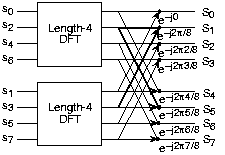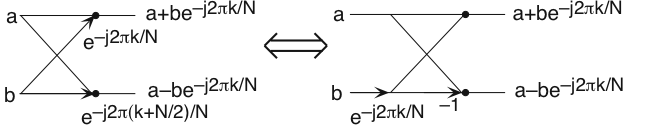# 3.2 Fast fourier transform (fft)  (Page 2/2)

Now for the fun. Because $N=2^{L}$ , each of the half-length transforms can be reduced to two quarter-length transforms, each of these to twoeighth-length ones, etc. This decomposition continues until we are left with length-2 transforms. This transform is quitesimple, involving only additions. Thus, the first stage of the FFT has $\frac{N}{2}$ length-2 transforms (see the bottom part of [link] ). Pairs of these transforms are combined by adding one to the other multiplied by a complexexponential. Each pair requires 4 additions and 2 multiplications, giving a total number of computations equaling $6·\frac{N}{4}=\frac{3N}{2}$ . This number of computations does not change from stage to stage.Because the number of stages, the number of times the length can be divided by two, equals $\log_{2}N$ , the number of arithmetic operations equals $\frac{3N}{2}\log_{2}N$ , which makes the complexity of the FFT $O(N\log_{2}N)$ .The initial decomposition of a length-8 DFT into the terms using even- and odd-indexed inputs marks the first phase ofdeveloping the FFT algorithm. When these half-length transforms are successively decomposed, we are left with the diagram shownin the bottom panel that depicts the length-8 FFT computation.

Doing an example will make computational savings more obvious. Let's look at the detailsof a length-8 DFT. As shown on [link] , we first decompose the DFT into two length-4 DFTs, with the outputs added and subtracted together in pairs.Considering [link] as the frequency index goes from 0 through 7, we recycle values fromthe length-4 DFTs into the final calculation because of the periodicity of the DFT output. Examining how pairs of outputsare collected together, we create the basic computational element known as a butterfly ( [link] ).The basic computational element of the fast Fourier transform is the butterfly. It takes two complex numbers, representedby a and b , and forms the quantities shown. Each butterfly requires onecomplex multiplication and two complex additions. By considering together the computations involving common output frequencies from the two half-length DFTs, we see that the twocomplex multiplies are related to each other, and we can reduce our computational work even further. By further decomposing thelength-4 DFTs into two length-2 DFTs and combining their outputs, we arrive at the diagram summarizing the length-8 fastFourier transform ( [link] ). Although most of the complex multiplies are quite simple(multiplying by $e^{-(i\frac{\pi }{2})}$ means swapping real and imaginary parts and changing their signs), let's count those forpurposes of evaluating the complexity as full complex multiplies. We have $\frac{N}{2}=4$ complex multiplies and $N=8$ complex additions for each stage and $\log_{2}N=3$ stages, making the number of basic computations $\frac{3N}{2}\log_{2}N$ as predicted.

Note that the ordering of the input sequence in the two parts of [link] aren't quite the same. Why not? How is the ordering determined?

The upper panel has not used the FFT algorithm to compute the length-4 DFTs while the lower one has. The ordering isdetermined by the algorithm.

Other "fast" algorithms were discovered, all of which make use of how many common factors the transformlength $N$ has. In number theory, the number of prime factors a given integer has measures how composite it is. The numbers 16 and 81 are highly composite (equaling $2^{4}$ and $3^{4}$ respectively), the number 18 is less so ( $2^{1}·3^{2}$ ), and 17 not at all (it's prime). In over thirty years of Fourier transform algorithm development, the originalCooley-Tukey algorithm is far and away the most frequently used. It is so computationally efficient that power-of-twotransform lengths are frequently used regardless of what the actual length of the data.

Suppose the length of the signal were $500$ ? How would you compute the spectrum of this signal using the Cooley-Tukeyalgorithm? What would the length $N$ of the transform be?

The transform can have any greater than or equal to the actual duration of the signal. We simply“pad” the signal with zero-valued samples until a computationally advantageous signal length results. Recallthat the FFT is an algorithm to compute the DFT . Extending the length of the signal this way merely means weare sampling the frequency axis more finely than required. To use the Cooley-Tukey algorithm, the length of theresulting zero-padded signal can be 512, 1024, etc. samples long.

what is variations in raman spectra for nanomaterials
I only see partial conversation and what's the question here!
what about nanotechnology for water purification
please someone correct me if I'm wrong but I think one can use nanoparticles, specially silver nanoparticles for water treatment.
Damian
yes that's correct
Professor
I think
Professor
what is the stm
is there industrial application of fullrenes. What is the method to prepare fullrene on large scale.?
Rafiq
industrial application...? mmm I think on the medical side as drug carrier, but you should go deeper on your research, I may be wrong
Damian
How we are making nano material?
what is a peer
What is meant by 'nano scale'?
What is STMs full form?
LITNING
scanning tunneling microscope
Sahil
how nano science is used for hydrophobicity
Santosh
Do u think that Graphene and Fullrene fiber can be used to make Air Plane body structure the lightest and strongest. Rafiq
Rafiq
what is differents between GO and RGO?
Mahi
what is simplest way to understand the applications of nano robots used to detect the cancer affected cell of human body.? How this robot is carried to required site of body cell.? what will be the carrier material and how can be detected that correct delivery of drug is done Rafiq
Rafiq
what is Nano technology ?
write examples of Nano molecule?
Bob
The nanotechnology is as new science, to scale nanometric
brayan
nanotechnology is the study, desing, synthesis, manipulation and application of materials and functional systems through control of matter at nanoscale
Damian
Is there any normative that regulates the use of silver nanoparticles?
what king of growth are you checking .?
Renato
What fields keep nano created devices from performing or assimulating ? Magnetic fields ? Are do they assimilate ?
why we need to study biomolecules, molecular biology in nanotechnology?
?
Kyle
yes I'm doing my masters in nanotechnology, we are being studying all these domains as well..
why?
what school?
Kyle
biomolecules are e building blocks of every organics and inorganic materials.
Joe
anyone know any internet site where one can find nanotechnology papers?
research.net
kanaga
sciencedirect big data base
Ernesto
Introduction about quantum dots in nanotechnology
what does nano mean?
nano basically means 10^(-9). nanometer is a unit to measure length.
Bharti
do you think it's worthwhile in the long term to study the effects and possibilities of nanotechnology on viral treatment?
absolutely yes
Daniel
how did you get the value of 2000N.What calculations are needed to arrive at it
Privacy Information Security Software Version 1.1a
Good
Got questions? Join the online conversation and get instant answers!ByBy Mistry Bhavesh